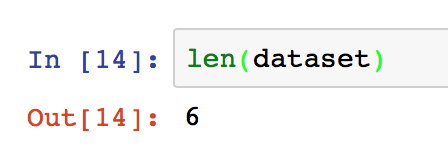# Dataset index problem

Recently, I’ve written a custom `Dataset` class to load my dataset. Here is what it looks like:

The folder name is 001 and the picture names are from 0 to 5. Here is my `mathDataset`:

``````class mathdataset(Dataset):
def __init__(self, root_dir, transform=None):
self.root_dir=root_dir
self.transform=transform

def __len__(self):
filenames=glob.glob(str(self.root_dir)+'/*.png')
return len(filenames)
def __getitem__(self,i):
img_name=str(self.root_dir)+'/'+str(i)+'.png'
im=Image.open(img_name)
image=im.convert('RGB')
if self.transform:
image = self.transform(image)
return image
``````

I’ve written the `__len__` and `__getitem__` as the doc suggested.

The problem came when I wanted to iterate through the dataset like this:

Then I checked the `len(dataset)`It was correct. And the last tensor in the `dataset` which size is `torch.Size([3, 224, 262])` correctly matched the picture `5.png`
So is there anything I can do like modify the `mathDataset` or rename the pictures to get the right output?

From the python docs

Note: `for` loops expect that an `IndexError` will be raised for illegal indexes to allow proper detection of the end of the sequence.

``````try: